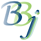# SQL — BIN() Function

BIN (intA,intB)

## Description

The BIN() function converts intA to a binary string that is intB bytes long.

The left-most bit is considered the "sign" bit. If "on" (1), the number is negative. Negative numbers are stored in twos complement (negative binary) notation.

Although the BIN() function returns a signed binary string, an overflow error is issued only if an unsigned overflow occurs. For example, BIN(255,1) and BIN(-1,1) both return \$FF\$ without an error.

The DEC() function performs the reverse operation of BIN() function. However, the DEC() function will always assume a signed binary value. DEC(BIN(255,1)) will return -1.

## Example

Select BIN(2,4) from mtable